# Using a number line to compare integers Online Quiz

Following quiz provides Multiple Choice Questions (MCQs) related to Using a number line to compare integers. You will have to read all the given answers and click over the correct answer. If you are not sure about the answer then you can check the answer using Show Answer button. You can use Next Quiz button to check new set of questions in the quiz.### Explanation

Step 1:

On the number line −36 lies to the left of 39. So −36 < 39

Step 2:

On number line### Explanation

Step 1:

On the number line 4 lies to the right of −7. So 4 > −7

Step 2:

On number line### Explanation

Step 1:

On the number line −13 lies to the left of 13. So −13 < 13

Step 2:

On number line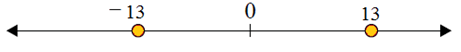### Explanation

Step 1:

On the number line 9 lies to the right of −3. So 9 > −3

Step 2:

On number line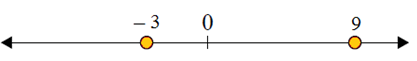### Explanation

Step 1:

On the number line −21 lies to the left of 32. So −21 < 32

Step 2:

On number line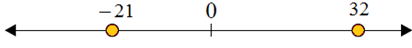### Explanation

Step 1:

On the number line 44 lies to the right of −56. So 44 > −56

Step 2:

On number line### Explanation

Step 1:

On the number line −17 lies to the left of 5. So −17 < 5

Step 2:

On number line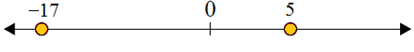### Explanation

Step 1:

On the number line −76 lies to the left of 53. So −76 < 53

Step 2:

On number line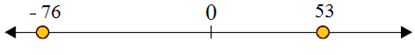### Explanation

Step 1:

On the number line −101 lies to the left of 99. So −101 < 99

Step 2:

On number line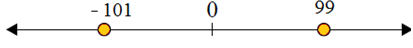### Explanation

Step 1:

On the number line −81 lies to the left of 63. So 63 > −81

Step 2:

On number line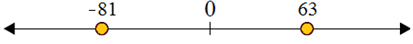using_number_line_to_compare_integers.htm